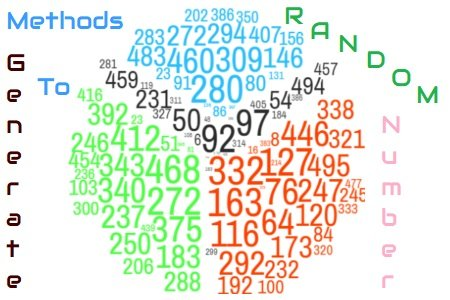# Random Number Generation in LoadRunnerIn the performance testing scenario, it is a good practice to use a random number where some arbitrary values are passed in the request. For example, you want to test an online registration functionality of a website. The registration form has some mandatory fields like Name, Age, Address etc. Keep rest aside, since the age field has an input range from 1 to 100, so a random variable having the same range can be used for testing purpose. That random variable should generate any number between 1 to 100 and make the scenario realistic. Now the question is how to generate a random number using VuGen script in LoadRunner?

Actually, there are three methods to generate a random number in LoadRunner:

1. Using rand() Function
2. By Random Parameter
3. By Date/Time Parameter

Following is the description of each method along with the example, Pros and Cons:

## 1. Using rand() Function

This is a pre-defined function in LoadRunner. rand() function generates a random value in the range 0 to 32767. Some basic mathematical operations and logic customize the range of random value. As per the above example, the age limit should be in between 1 to 100. Hence the following code will generate the required value:

``````Action()
{
int randNumber;
randNumber = rand()%100 + 1;  //Generate Random Number between 1 to 100
lr_output_message("Random Number is %d", randNumber);
return 0;
}

Output:
Starting action Action.
Action.c(5): Random Number is 26
Ending action Action.``````

### Pros:

1. The random number can be generated using the basic function of maths.
2. Ease of generating the number using offset. In the above code ‘1’ is an offset which gets added in the generated random numbers every time.
3. A negative number can also be generated by subtracting the offset.

### Cons:

1. The scope of the generated random number is throughout the iteration. If a different random number needs to be passed within the same iteration then this function needs to be recalled, so that another random number can be generated.
2. The format cannot be specified.

## 2. By Random Parameter:

This is the easiest way to generate a random number in LoadRunner script. In this method, a parameter needs to be defined in the ‘Parameters’ by selecting ‘Parameter Type’ as ‘Random Number’.

The value can be fetched by using the parameter name as shown below:

``````Action()
{
lr_output_message("Random Number is %d", atoi(lr_eval_string("{randParam}")));
return 0;
}

Output:
Starting action Action.
Action.c(3): Random Number is 77
Ending action Action.``````

### Pros:

1. The number can be generated by simply defining a parameter of type ‘Random Number’.
2. Ease of specifying the range.
3. No need to write mathematical logic.
4. Number format can be easily defined.
5. A negative number can also be generated by using a minus sign (-) in the ‘Number Format’ field.
6. The scope of the random number can be easily defined by using ‘Update value on’ setting. A new random value can be generated at each iteration, each occurrence and once.
7. Support to generate x digit(s) value.

### Cons:

1. No option for offset.

## 3. By Date/Time Parameter:

With the help of the combination of date and time, a random number can be generated. In this method, a parameter of type Date/Time needs to be defined and the number format is created by using the combination of year, month, day, hour, minute and second.

The value can be fetched by using the parameter name as shown below:

``````Action()
{
lr_output_message("Random Number is %d", atoi(lr_eval_string("{randParam}")));
return 0;
}

Output:
Starting action Action.
Action.c(3): Random Number is 171007
Ending action Action.``````

### Pros:

1. The random number can be generated easily, but needs to take care of special character like /, – etc. (do not include them)
2. No need to write mathematical logic
3. A negative number can be generated by using a minus sign (-) in the ‘Date/Time Format’ field
4. The scope of the random number can be easily defined by using ‘Update value on’ setting. A new random value can be generated at each iteration, each occurrence and once
5. Support to generate x digit(s) value
6. An offset can be defined

### Cons:

1. The range cannot be specified.
2. The number format is defined using the tokens of date and time only.
3. It does not allow to specify the millisecond format (without a dot).
4. Not suitable to generate small digit numbers.

You can choose any of the above-mentioned methods to generate a random number in the VuGen script.

You may be interested: